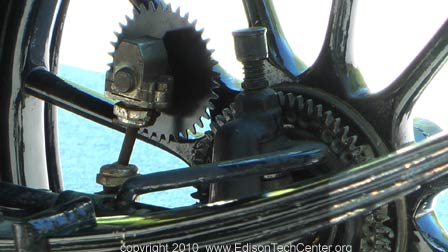# Pi Day Quiz 2016

Its time for the annual Edison Tech Center Pi Day Quiz!

2016 Edison Tech Center Pi Day Quiz

1) The Marchant electromechanical calculator consists of several interlocking gears which are driven by an electric motor. If all the gears have a diameter of one unit, and each gear has ten teeth, what’s the total distance the gears travel for the following calculation:
999+1001

2) The Marchant electromechanical calculator consists of several interlocking gears which are driven by an electric motor. If all the gears have a diameter of one unit, and each gear has ten teeth, what’s the total distance the gears travel for the following calculation:
999 multiplied by 1001

3) In a Synchronous AC motor, the R.P.M.s are ideally equal to (120 X Freq) divided by the number of magnetic poles.
How fast is the outer material of a 1.5” diameter rotor traveling, when energized at 60 Hz on a synchronous, 4 pole motor?
– Question by Shane Pickett, Nidec Drive Systems Engineer

4) The Marchant electromechanical calculator consists of several interlocking gears which are driven by an electric motor. If all the gears have a diameter of one unit, and each gear has ten teeth, what’s the total distance the gears travel for the following calculation:
1001 minus 999

5) A tire company has twenty stacks of tires up to eight tires high, of seven different diameters. What is the most efficient arrangement of the tires to be able to access any tire?
– Question by Gene Bills

6) A machine to weave canvas sacks uses 6 spools of thread evenly placed around a rotating circular spindle. How much more thread is used for a canvas sack with twice the diameter?

7) How much more volume does a canvas sack with twice the diameter of a smaller canvas sack have?